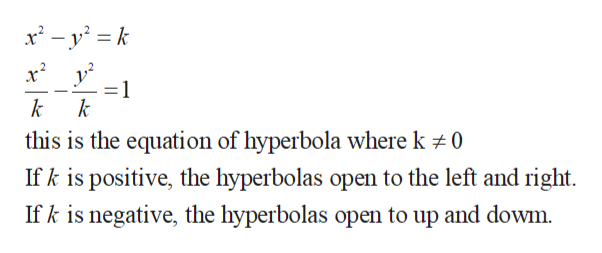# Let f(x,y)= x2-y2a) On the set of xy-axes, draw the level curves L(x,y) = k for k= 1,2,3. On another set of axes, draw the level curves f(x,y)= k for k=1,2,3. (Do this part by hand)b) How do the contour maps in part (a) reflect the fact that L is the linear approximation to f at the point (2,1)? Explain briefly in words.

Question
105 views

Let f(x,y)= x2-y2

a) On the set of xy-axes, draw the level curves L(x,y) = k for k= 1,2,3. On another set of axes, draw the level curves f(x,y)= k for k=1,2,3. (Do this part by hand)

b) How do the contour maps in part (a) reflect the fact that L is the linear approximation to f at the point (2,1)? Explain briefly in words.

check_circle

Step 1

The level curves f (x, y) = k are just the traces of the graph of f in the horizontal plane z = k projected down to the xy-plane.

Let f (x, y) = x2 – y2, now the level curve k = x2 – y2

For k = 1

Consider the curve k = x2 – y2help_outlineImage Transcriptionclose=1 k k this is the equation of hyperbola where k 0 Ifk is positive, the hyperbolas open to the left and right. If k is negative, the hyperbolas open to up and dowm. fullscreen
Step 2

Now suppose for k =1

The obtained equation is equation of hyperbola and open up to the left and right, shown in the below diagram:

Step 3

For k = 1, 2, 3, …. The obtained curve is sh...

### Want to see the full answer?

See Solution

#### Want to see this answer and more?

Solutions are written by subject experts who are available 24/7. Questions are typically answered within 1 hour.*

See Solution
*Response times may vary by subject and question.
Tagged in

### Calculus